EXAMPLE 4: THE ANGULAR IMPULSE-MOMENTUM RELATION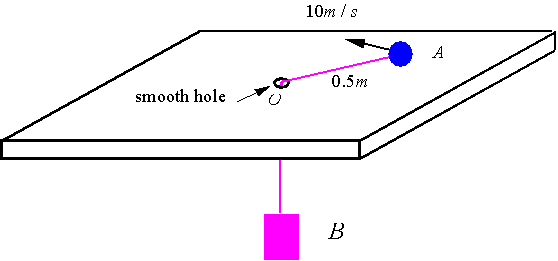Particle A of mass 1 kg moves on a smooth horizontal surface and is connected by a string to particle B. B has a mass of 2 kg. Particle B is released from rest at time t=0 when particle A has a circumferential velocity of 10 m/s and a radius of 0.5 m. Write the equation of motion of the system.

Solution: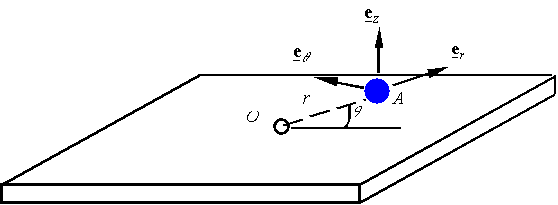Particle A: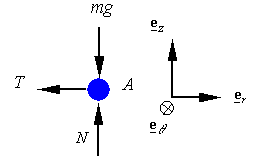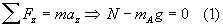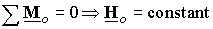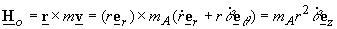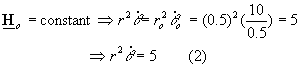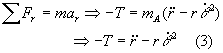Particle B: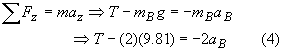Kinematics relation: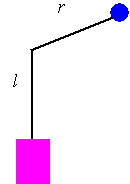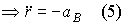# Add (3) and (4) and substitute (5) to get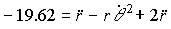Substitution from (2) gives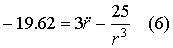Equation (2) and (6) are the equation of motion for the system.